PSABHXC - Elementary Algebra Accuplacer ASSISTments Evaluation - B

Factor:Select one:

Solve the system of equations.Select one:

Multiply.Select one:

Graph the linear inequality.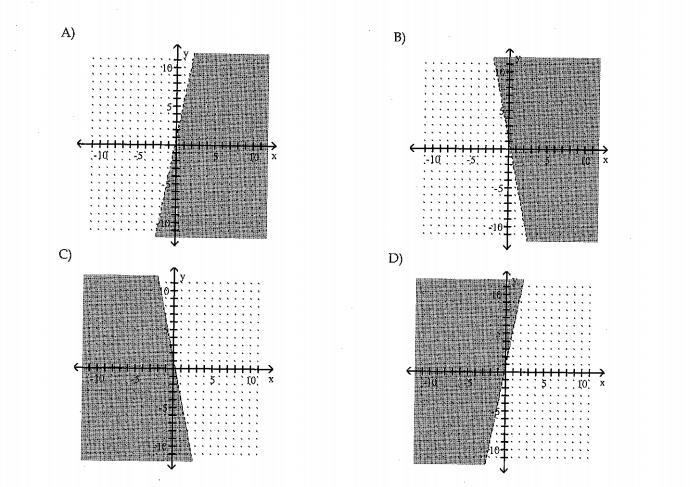Select one:

A 30% iodine solution is mixed with a 60% iodine solution to produce 3 gallons of 40% Iodine solution. How many gallons of each solution are needed?

Select one:

In the right triangle shown, find the length of the missing side.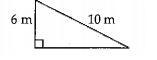Select one:

Divide: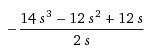Select one:
 Divide: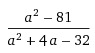by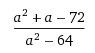Select one:

Simplify, assuming all variables represent non negative real numbers.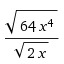Select one:

Simplify, assuming all variables represent non negative real numbers.Select one:
Determine whether 7 is a solution of the equation 2y + 5(y-4) = 29.
Select one:

(x+10)(x-9) =  -18

Select one:
A car rental buisness rents a compact car at a daily rate of \$34.20 plus 20 cents per mile. Mike can afford to spend \$51 on the rental car for one day. How many miles can he drive and stay within his budget?
Select one:
Simplify: -9 + 4(-2) -11
Select one: